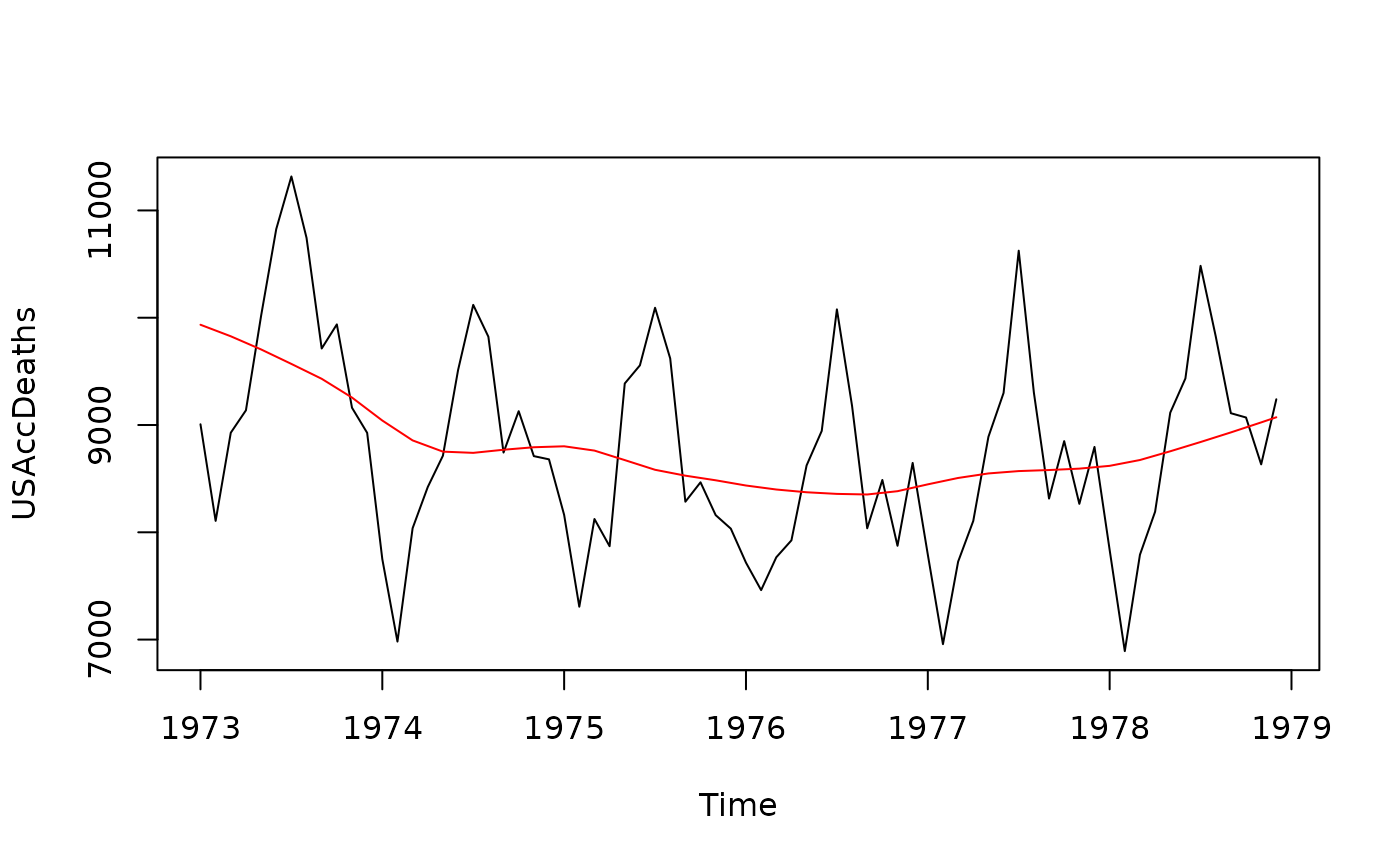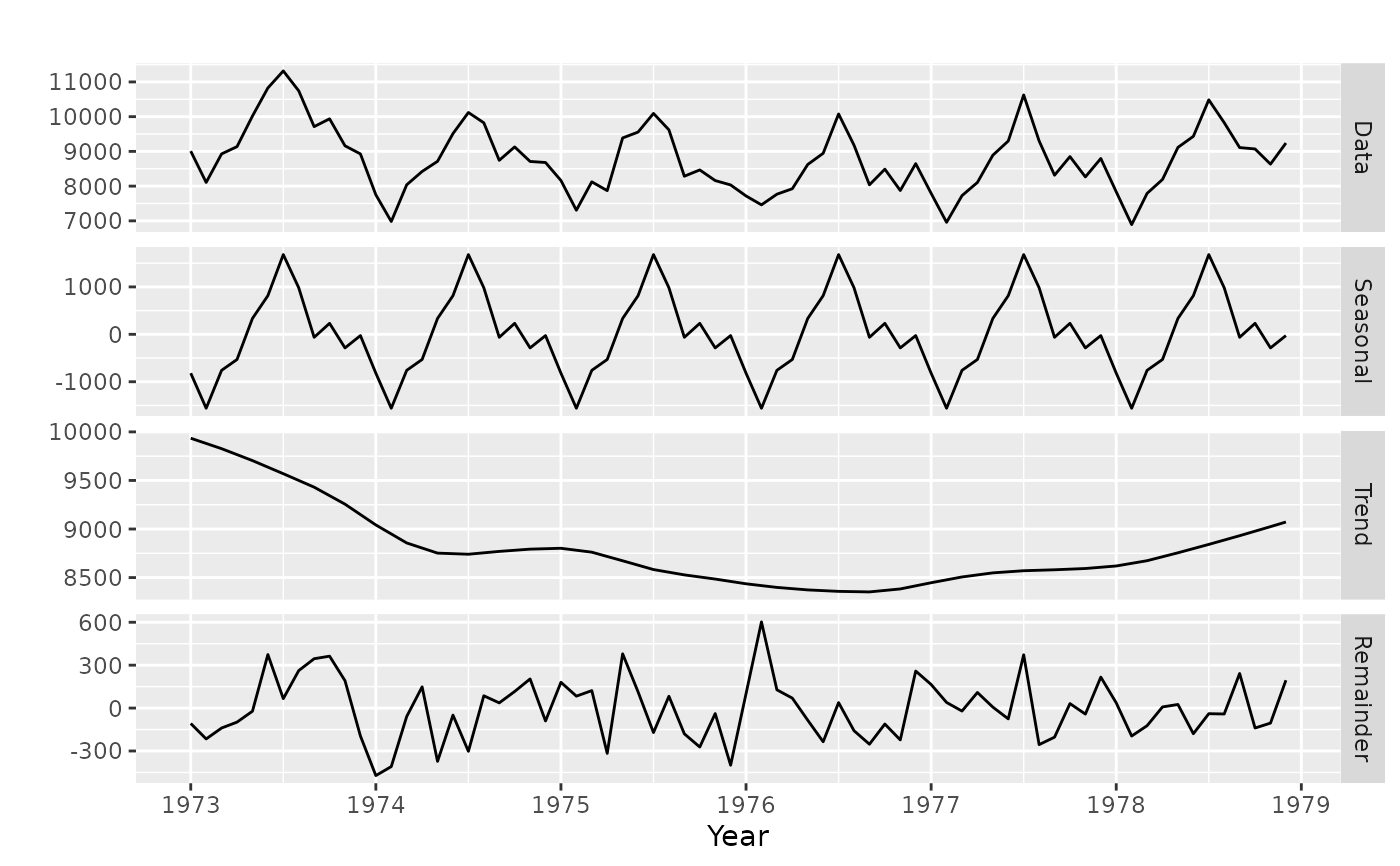Returns a univariate time series equal to either a seasonal component, trend-cycle component or remainder component from a time series decomposition.

seasonal(object)

trendcycle(object)

remainder(object)

## Arguments

object Object created by decompose, stl or tbats.

## Value

Univariate time series.

stl, decompose, tbats, seasadj.

Rob J Hyndman

## Examples

plot(USAccDeaths)
fit <- stl(USAccDeaths, s.window="periodic")
lines(trendcycle(fit),col="red")library(ggplot2)
autoplot(cbind(
Data=USAccDeaths,
Seasonal=seasonal(fit),
Trend=trendcycle(fit),
Remainder=remainder(fit)),
facets=TRUE) +
ylab("") + xlab("Year")